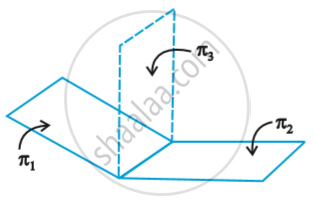# Plane - Plane Passing Through the Intersection of Two Given Planes

#### notes

Let π_1 and π_2 be two planes with equations vec r  . hat n _1 = d_1 and vec r . hat n _2 = d_2 respectively.  The position vector of any point on the line of intersection must satisfy both the equations fig.If vec t  is the position vector of a point on the line , then
vec t . hat n_1 = d_1 and vec t . hat n _2 = d_2
Therefore , for all real values of  λ, we have
vec t . (hat n _1 + lambda hat n_2) = d_1 + lambda d_2
Since vec t is arbitrary, it satisfies for any point on the line.
Hence , the equation vec r . (vec n_1 + lambda vec n_2) = d_1 + lambda d_2   represents a plane π_3 which is such  that if any vector  vec r satisfies both the equations π_1 and π_2, it also satisfies the equation π_3 i.e., any plane passing through the intersection of the planes
vec r . vec n_1 = d_1 and vec r . vec n_2 = d_2
has the equation  vec r . (vec n_1 + lambda vec n_2) = d_1 + lambda d_2    ...(1)

Cartesian form:
In Cartesian system, let  vec n_1 = A_1 hat i + B_2 hat j + C_1 hat k
vec n_2 = A_2 hat i + B_2 hat j + C _2 hat k
and vec r = x hat i + y hat j + z hat k
Then (1) becomes
x (A_1 + lambda A_2) + y(B_1 + lambda B_2) + z(C_1 +lambda C_2) = d_1 + lambda d_2
or (A_1x +B_1y + C_1z -d_1) + lambda (A_2x + B_2y + C_2z -d_2) = 0     ..(2)
which is the required Cartesian form of the equation of the plane passing through the intersection of the given planes for each value of λ.

If you would like to contribute notes or other learning material, please submit them using the button below.